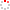#CodeStepByStep## unflatten

Language/Type: C++ Grid Vector collections

Write a method named `unflatten` that accepts three parameters: a reference to a `Vector` of integers, and a number of rows and columns. Your method should convert the vector into a `Grid` with the given number of rows and columns, where values are transferred in row-major order into the grid. For example, if the given vector is declared:

```Vector<int> v {3, 8, 12, 2, 9, 17, 43, -8, 46, 203, 14, 97};
```

Then the call of `unflatten(v, 4, 3)` should return the following grid:

```{{  3,   8,  12},
{  2,   9,  17},
{ 43,  -8,  46},
{203,  14,  97}}
```

The call of `unflatten(v, 6, 2)` should return the following grid:

```{{  3,   8},
{ 12,   2},
{  9   17},
{ 43,  -8},
{ 46, 203},
{ 14,  97}}
```

If the vector's contents do not fit exactly into a grid of the given dimensions, your method should throw an `int` exception. Your code should work for a vector of any size. Your method should not modify the vector that is passed in.

Type your C++ solution code here:

This is a function exercise. Write a C++ function as described. Do not write a complete program; just the function(s) above.